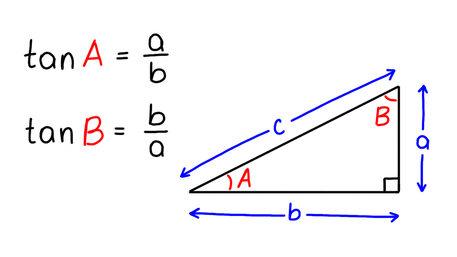VIEW IN FULL SCREEN

# AQA A-level Maths TangentTangent of an angle is a ratio between the side opposite to the angle and the side adjacent to the angle.Tangent is one of three trigonometric functions of an angle.tan(x) is found by calculating the ratio of two sides of a right-angled triangle, the opposite and the adjacent.The diagram shows a tangent graph.# ✅

Find a if tanA = 1.2 and b = 15.# ✅

Find tanB if a = 0.2 m and b = 1 m.# ✅

Use your calculator to find the value of tan(0).# ✅

tan(xº) = 1. Given that x is an angle in a triangle, what is the value of x?# ✅

Use your calculator to find the value of tan(40º).# ✅

Give the equation / ratio used to find the value of tan(x).# ✅

If x is an angle in a triangle with opposite = 3 and adjacent = 6, what is the value of tan x?# ✅

A triangle has hypotenuse 18, opposite 12 and adjacent 6√5. What is the value of tan x where x is the angle in the triangle, and hence what is the value of x?# ✅

At what point does the tangent graph cross the y axis?# ✅

Write down the x coordinate of point B on the tangent graph.Have you found the questions useful?